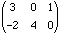Date: Thu, 30 Apr 1998 00:05:53 -0400
Subject: Mathematical Arrays

Good Day,

Could someone please explain what a mathematical or arithmetical array is? The array in question is used in grade three math in Ontario. I would also like to know what its purpose is. My E-mail address is;
glanctot@mail.on.rogers.wave.ca. Thank you for your time.

Regards
Gene

Hi Gene,
A mathematical array is a rectangular arrangement of objects. The Saskatchewan curriculum says "an array is an orderly arrangement of objects in columns and rows". The objects are quite often numbers. For example,

 3 0 1 -2 4 0

is a 2 by 3 array (rows are always mentioned before columns). Mathematicians usually use the word matrix for an array and would write the matrix above asAn array is often a useful way to keep a collection of things. My gradebook is an array of with one row for each student and one column for each homework assignment or test. This array is not composed entirely of numbers since the first column contains student names and the first row identifies the test or assignment.

As an example you might create a "pet array" for five students.

 dogs cats hampsters fish Mary Bob Fred Jane Laura

If the students fill in the table with the number of pets they have then by adding across the "Jane" row you see how many pets Jane has. By adding down the "dogs" column you see how many dog pets there are, and by counting the number of entries in the "cat" column that are not zeros, you see how many of the students have cats as pets.

I hope this helps,
Harley

Go to Math Central